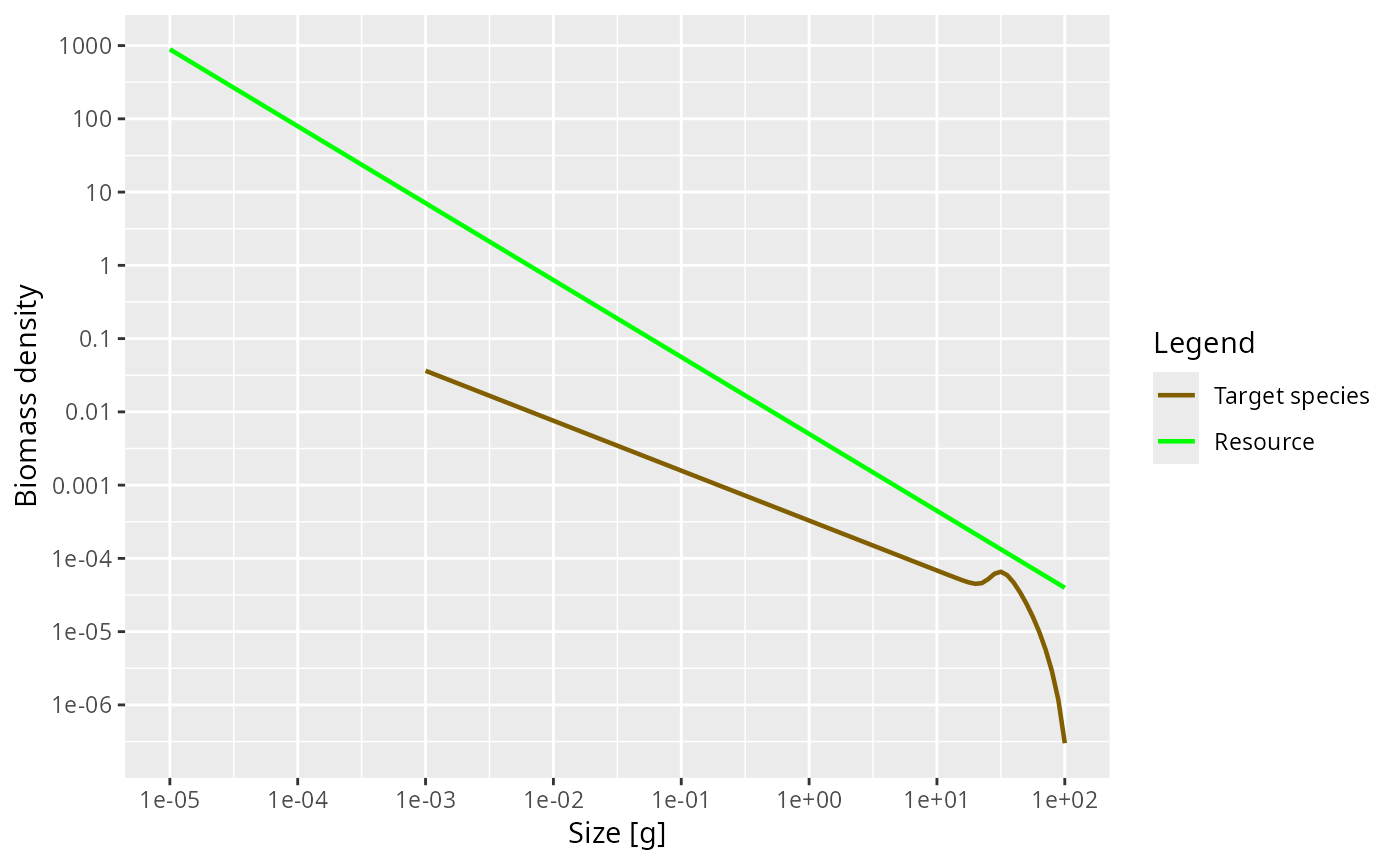This functions creates a MizerParams object with a single species. This species is embedded in a fixed power-law community spectrum $$N_c(w) = \kappa w^{-\lambda}$$ This community provides the food income for the species. Cannibalism is switched off. The predation mortality arises only from the predators in the power-law community and it is assumed that the predators in the community have the same feeding parameters as the foreground species. The function has many arguments, all of which have default values.

newSingleSpeciesParams(
species_name = "Target species",
w_inf = 100,
w_min = 0.001,
eta = 10^(-0.6),
w_mat = w_inf * eta,
no_w = log10(w_inf/w_min) * 20 + 1,
n = 3/4,
p = n,
lambda = 2.05,
kappa = 0.005,
alpha = 0.4,
k_vb = 1,
beta = 100,
sigma = 1.3,
f0 = 0.6,
fc = 0.25,
ks = NA,
gamma = NA,
ext_mort_prop = 0,
reproduction_level = 0,
R_factor = deprecated()
)

## Arguments

species_name A string with a name for the species. Will be used in plot legends. Asymptotic size of species Egg size of species Ratio between maturity size w_mat and asymptotic size w_inf. Default is 10^(-0.6), approximately 1/4. Ignored if w_mat is supplied explicitly. Maturity size of species. Default value is eta * w_inf. The number of size bins in the community spectrum. These bins will be equally spaced on a logarithmic scale. Default value is such that there are 20 bins for each factor of 10 in weight. Scaling exponent of the maximum intake rate. Scaling exponent of the standard metabolic rate. By default this is equal to the exponent n. Exponent of the abundance power law. Coefficient in abundance power law. The assimilation efficiency. The von Bertalanffy growth parameter. Preferred predator prey mass ratio. Width of prey size preference. Expected average feeding level. Used to set gamma, the coefficient in the search rate. Ignored if gamma is given explicitly. Critical feeding level. Used to determine ks if it is not given explicitly. Standard metabolism coefficient. If not provided, default will be calculated from critical feeding level argument fc. Volumetric search rate. If not provided, default is determined by get_gamma_default() using the value of f0. The proportion of the total mortality that comes from external mortality, i.e., from sources not explicitly modelled. A number in the interval [0, 1). A number between 0 an 1 that determines the level of density dependence in reproduction, see setBevertonHolt().Use reproduction_level = 1 / R_factor instead.

## Value

An object of type MizerParams

## Details

In addition to setting up the parameters, this function also sets up an initial condition that is close to steady state, under the assumption of no fishing.

Other functions for setting up models: newCommunityParams(), newMultispeciesParams(), newTraitParams()
params <- newSingleSpeciesParams()